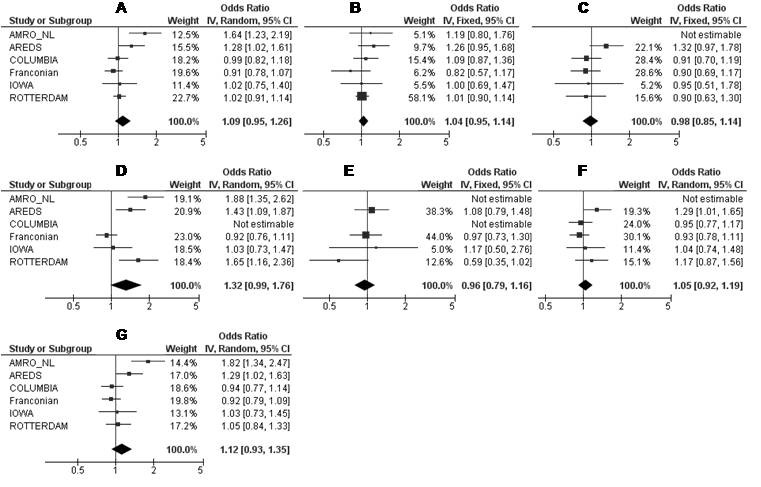Figure 4 of Baas, Mol Vis 2012; 18:657-674.

Figure 4. Meta-analysis of rs841853 for its association with all AMD cases (A), early (B), GA (C), CNV (D), mixed (E), CNV + mixed (F), and late AMD (G) cases in six independent study populations. AMD=age-related macular degeneration; GA=geographic atrophy; CNV=choroidal neovascularization; Mixed cases (combination of GA + CNV). Odds ratios (ORs, squares) and 95% confidence intervals (CI; horizontal lines) are presented for each study. Also shown is the black diamond of the summary OR using either the additive Mantel-Haenszel fixed effects model when heterogeneity was absent (I2 <25%) or the additive DerSimonian and Laird random effects model when heterogeneity was present (I2 >25%). Heterogeneity between studies was tested using Cochran's Q statistic and the I-square statistic for inconsistency.